# Temporal Grid

## Definition

In the context of a Credit Network model, a Temporal Grid denotes the specification of

## Usage

In the simplest case, future cashflows of credit asset and liabilities that are part of the credit network are considered at a set of given timepoints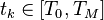$t_k \in [T_0,T_M]$.

The modelling period spans the time interval from the current date (as-off date)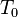$T_0$ till the end of the calculation period (e.g., expected life / longest tenor)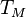$T_M$ of all credit assets and/or liabilities).

NB: For brevity we may drop the M index and refer to $T$ instead and similarly indicate a timepoint by its index k instead of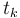$t_k$.

## Grid Variables

All variables represented on the grid are either state or flux (flow) variables. State variables are associated (e.g represent state) with a single timepoint$t_k$ whereas flow variables are associated with a period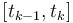$[t_{k-1}, t_k]$.

## Issues and Challenges

• When a temporal grid is selected to be coarse (e.g. annual) all input data must be appropriately mapped to timepoints/periods.